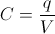## What is an electric field?

An electric field is a special state that exists in the space surrounding an electrically charged particle. This special state affects all charged particles placed in the electric field. The true nature of electric fields, as well as the true nature of an electric charge is still unknown to scientists, but the effects of an electric field can be measured and predicted using known equations.
Just like a magnet creates an invisible magnetic field around it, which can be detected by placing a second magnet in its field and measuring the attractive or repulsive force acting on the magnets, electric charges create an electric field which can be detected by using a test charge. When a test charge is placed inside an electric field, an attractive or repulsive force acts upon it. This force is called the Coulomb force. In fact, magnetic and electric fields are not entirely separate phenomena. A magnetic field that changes with time creates – or “induces” an electric field, while a moving electric field induces a magnetic field as a direct consequence of the movement. Because these two fields are so tightly connected, the magnetic and electric fields are combined into one, unified, electromagnetic field.

## Electric field definition

The electric field can be defined as a vector field which describes the relationship between the charge of a test particle introduced in the field and the force exerted upon this charged test particle.Where E is the electric field, F is the force exerted on the test particle introduced into the field and q is the charge of the test particle. The unit for electric field is volts per meter [V·m-1] or newtons per coulomb [N·C-1].

## The application of electric field in capacitors

Electromagnetism is a science which studies static and dynamic charges, electric and magnetic fields and their various effects. Capacitors are devices which store electrical potential energy using an electric field. As such, capacitors are governed by the rules of electromagnetism. This article will define and outline some of the terms which are needed to understand the workings of capacitors. In this article, it will be considered that the electric field is uniform in all points in space.

### Electric potential energy

Electric potential energy is the potential energy of a charged particle in an electric field which results from the Coulomb force acting on the particle. It is defined as the negative of the amount of work needed to bring the particle from a reference point (often infinitely far away) to the point in space where the electric potential energy is measured. The unit for electric potential energy is a joule [J], the same unit used for the amount of work in physics.

### Electric potential

Electric potential, also called the electric field potential, is the amount of electric potential energy that a charged particle would have at a certain point in space. The voltage, also called potential difference between two points in space is the difference of the electric potentials of those two points. The unit used for electric potential is a volt [V], named after the italian physicist Alessandro Volta. The same unit is used for voltage. The electric potential between two points in an uniform field is the negative of the field intensity difference between those two points.

### Electric field strength

In a simple parallel-plate capacitor, a voltage applied between two conductive plates creates a uniform electric field between those plates. The electric field strength in a capacitor is directly proportional to the voltage applied and inversely proportional to the distance between the plates. This factor limits the maximum rated voltage of a capacitor, since the electric field strength must not exceed the breakdown field strength of the dielectric used in the capacitor. If the breakdown voltage is exceeded, an electrical arc is generated between the plates. This electric arc can destroy some types of capacitors instantly. The standard unit used for electric field strength is volts per meter [V·m-1].

### Capacitance

Capacitance represents the ability of a body to store electrical charge. This ability is used in capacitors to store electrical energy by sustaining an electric field. When voltage is applied to a capacitor, a certain amount of positive electric charge (+q) accumulates on one plate of the capacitor, while an equal amount of negative electric charge (-q) accumulates on the other plate of the capacitor. It is defined as:where C is the capacitance, q is the amount of charge accumulated on the plates and V is the voltage across the two plates of the capacitor.
Capacitance is a function of the capacitor’s geometry. Factors such as the area of the plates, the distance between the plates and the dielectric constant of the dielectric used in the construction of the capacitor all influence the resulting capacitance. In a simple parallel plate, the electrical capacitance is directly proportional to the area of the plates and the dielectric constant, while it is inversely proportional to the distance between the plates. The unit used for capacitance is a Farad [F], named after Michael Faraday, who was the pioneer of research in electricity and magnetism.

### Energy stored on a capacitor

Capacitors are devices which are used to store electrical energy in a circuit. The energy supplied to the capacitor is stored in the form of an electric field which is created between the plates of a capacitor. When the voltage is applied across a capacitor, a certain amount of charge accumulates on the plates. The energy stored on the capacitor is:where W is the energy stored, C is the capacitance and V is the voltage applied across the capacitor.